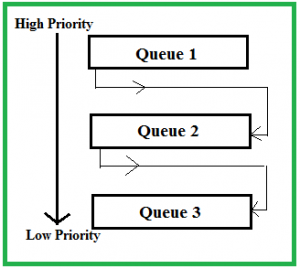Related Articles
Multilevel Feedback Queue Scheduling (MLFQ) CPU Scheduling
• Difficulty Level : Easy
• Last Updated : 14 Aug, 2020

Prerequisite – CPU Scheduling, Multilevel Queue Scheduling
This Scheduling is like Multilevel Queue(MLQ) Scheduling but in this process can move between the queues. Multilevel Feedback Queue Scheduling (MLFQ) keep analyzing the behavior (time of execution) of processes and according to which it changes its priority.Now, look at the diagram and explanation below to understand it properly.Now let us suppose that queue 1 and 2 follow round robin with time quantum 4 and 8 respectively and queue 3 follow FCFS.One implementation of MFQS is given below –

1. When a process starts executing then it first enters queue 1.
2. In queue 1 process executes for 4 unit and if it completes in this 4 unit or it gives CPU for I/O operation in this 4 unit than the priority of this process does not change and if it again comes in the ready queue than it again starts its execution in Queue 1.
3. If a process in queue 1 does not complete in 4 unit then its priority gets reduced and it shifted to queue 2.
4. Above points 2 and 3 are also true for queue 2 processes but the time quantum is 8 unit.In a general case if a process does not complete in a time quantum than it is shifted to the lower priority queue.
5. In the last queue, processes are scheduled in FCFS manner.
6. A process in lower priority queue can only execute only when higher priority queues are empty.
7. A process running in the lower priority queue is interrupted by a process arriving in the higher priority queue.

Well, above implementation may differ for example the last queue can also follow Round-robin Scheduling.

Problems in the above implementation – A process in the lower priority queue can suffer from starvation due to some short processes taking all the CPU time.
Solution – A simple solution can be to boost the priority of all the process after regular intervals and place them all in the highest priority queue.

What is the need of such complex Scheduling?

• Firstly, it is more flexible than the multilevel queue scheduling.
• To optimize turnaround time algorithms like SJF is needed which require the running time of processes to schedule them. But the running time of the process is not known in advance. MFQS runs a process for a time quantum and then it can change its priority(if it is a long process). Thus it learns from past behavior of the process and then predicts its future behavior.This way it tries to run shorter process first thus optimizing turnaround time.
• MFQS also reduces the response time.

Example –
Consider a system which has a CPU bound process, which requires the burst time of 40 seconds.The multilevel Feed Back Queue scheduling algorithm is used and the queue time quantum ‘2’ seconds and in each level it is incremented by ‘5’ seconds.Then how many times the process will be interrupted and on which queue the process will terminate the execution?

Solution –
Process P needs 40 Seconds for total execution.
At Queue 1 it is executed for 2 seconds and then interrupted and shifted to queue 2.
At Queue 2 it is executed for 7 seconds and then interrupted and shifted to queue 3.
At Queue 3 it is executed for 12 seconds and then interrupted and shifted to queue 4.
At Queue 4 it is executed for 17 seconds and then interrupted and shifted to queue 5.
At Queue 5 it executes for 2 seconds and then it completes.
Hence the process is interrupted 4 times and completes on queue 5.

1. It is more flexible.
2. It allows different processes to move between different queues.
3. It prevents starvation by moving a process that waits too long for lower priority queue to the higher priority queue.